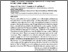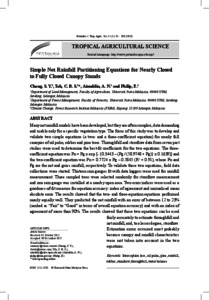# Simple net rainfall partitioning equations for nearly closed to fully closed canopy stands

## Citation

Chong, Siam Yee and Teh, Christopher Boon Sung and Nuruddin, Ahmad Ainuddin and M. P. Philip, Elizabeth (2018) Simple net rainfall partitioning equations for nearly closed to fully closed canopy stands. Pertanika Journal of Tropical Agricultural Science, 41 (1). pp. 81-100. ISSN 1511-3701; ESSN: 2231-8542

## Abstract

Many net rainfall models have been developed, but they are often complex, data demanding and usable only for a specific vegetation type. The focus of this study was to develop and validate two simple equations (a two- and a three-coefficient equation) for nearly full canopies of oil palm, rubber and pine trees. Throughfall and stemflow data from seven past studies were used to determine the best-fit coefficients for the two equations. The three-coefficient equation was Pn = Pg x exp [- {0.3443 – (Pg / (58.9748 + Pg)} x 0.1639)] and the two-coefficient equation was Pn = 0.7724 x Pg – 0.5845 (R2 = 0.91), where Pn and Pg are the net and gross rainfall, respectively. To validate these two equations, field data collections were started. Thirteen rain gauges fit with data loggers were used for rainfall measurement. Three sampled trees were selected randomly for stemflow measurement and one rain gauge was installed at a nearby open area. Two error indices were used as a goodness-of-fit measure for equation accuracy: index of agreement and normalised mean absolute error. The results showed that the two- and three-equation equations performed nearly equally well. They predicted the net rainfall with an error of between 12 to 23% (ranked as "Fair" to "Good" in terms of overall equation accuracy) and with an index of agreement of more than 90%. The results showed that these two equations can be used fairly accurately to estimate throughfall and net rainfall, and, to a lesser degree, stemflow. Estimation errors occurred most probably because canopy and rainfall characteristics were not taken into account in the two equations.Preview
Text
05 JTAS-0484-2012-5thProof.pdfView Item